# CAT 2019 Question Paper | Quants Slot 1

###### CAT Previous Year Paper | CAT Quants Questions | Question 12

This is a very time consuming question from CAT 2019 when done in an algebraic manner. It involves a lot of formulas and manipulation of variables. However if you observe the CAT previous year paper, you will observe there are usually questions that can be solved using a mix of your math intuition and option choices. This is one such question. Try both the conventional method & see if you are able to spot the quicker method as well.

Question 12 : The product of two positive numbers is 616. If the ratio of the difference of their cubes to the cube of their difference is 157 : 3, then the sum of the two numbers is

1. 50
2. 85
3. 95
4. 58

## Best CAT Coaching in Chennai

#### CAT Coaching in Chennai - CAT 2022Limited Seats Available - Register Now!

Let the two numbers be a and b
From the question, we know
a x b = 616
and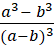=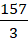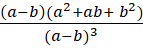=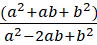=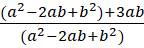=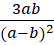=- 1 =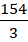=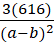=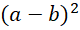= 3 x 3 x 4 = 36
a - b = 6, ab = 616
616 can be rewritten as 22 x 28, where 28 - 22= 6 using trial and error
So, a + b = 28 + 22 = 50

The question is "The product of two positive numbers is 616. If the ratio of the difference of their cubes to the cube of their difference is 157 : 3, then the sum of the two numbers is "

##### Hence, the answer is 50

Choice A is the correct answer.

###### CAT Coaching in ChennaiCAT 2023

Classroom Batches Starting Now! @Gopalapuram

###### Best CAT Coaching in Chennai Introductory offer of 5000/-

Attend a Demo Class

##### Where is 2IIM located?

2IIM Online CAT Coaching
A Fermat Education Initiative,
58/16, Indira Gandhi Street,
Kaveri Rangan Nagar, Saligramam, Chennai 600 093

##### How to reach 2IIM?

Mobile: (91) 99626 48484 / 94459 38484
WhatsApp: WhatsApp Now
Email: info@2iim.com